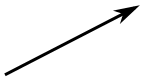# Complex PlaneNo, not that complex plane ... ... this complex plane:A plane for complex numbers!

(Also called an "Argand Diagram")

## Real and Imaginary make Complex

A Complex Number is a combination of a Real Number and an Imaginary Number:

A Real Number is the type of number we use every day.

Examples: 12.38, ½, 0, −2000

When we square a Real Number we get a positive (or zero) result:

22 = 2 × 2 = 4
12 = 1 × 1 = 1
02 = 0 × 0 = 0

What can we square to get −1?

?2 = −1

Squaring −1 does not work because multiplying negatives gives a positive: (−1) × (−1) = +1, and no other Real Number works either.

So it seems that mathematics is incomplete ...

... but we can fill the gap by imagining there is a number that, when multiplied by itself, gives −1
(call it i for imaginary):

i2 = −1

An Imaginary Number, when squared gives a negative result.

Examples: 5i, -3.6i, i/2, 500i

And together:

A Complex Number is a combination of a Real Number and an Imaginary Number

Examples: 3.6 + 4i, −0.02 + 1.2i, 25 − 0.3i, 0 + 2i

## Putting a Complex Number on a Plane

You may be familiar with the number line:But where do we put a complex number like 3+4i ?

Let's have the real number line go left-right as usual, and have the imaginary number line go up-and-down:

 We can then plot a complex number like 3 + 4i : 3 units along (the real axis), and 4 units up (the imaginary axis).And here is 4 - 2i : 4 units along (the real axis), and 2 units down (the imaginary axis).And that is the complex plane:

• complex because it is a combination of real and imaginary,
• plane because it is like a geometric plane (2 dimensional).

## Whole New World

Now let's bring the idea of a plane (Cartesian coordinates, Polar coordinates, Vectors etc) to complex numbers.

It will open up a whole new world of numbers that are more complete and elegant, as you will see.

## Complex Number as a Vector

We can think of a complex number as a vector.This is a vector.
It has magnitude (length) and direction.

 And here is the complex number 3 + 4i as a Vector:You can add complex numbers as vectors, too:

 To add the complex numbers 3 + 5i and 4 − 3i : add the real numbers, and add the imaginary numbers separately, like this: (3 + 5i) + (4 − 3i) =(3 + 4)+ (5 − 3)i =7+ 2i## Polar Form

 Let's use 3 + 4i again:Here it is in polar form:So the complex number 3 + 4i can also be shown as distance (5) and angle (0.927 radians).

Let's see how to convert from one form to the other using Cartesian to Polar conversion:

### Example: the number 3 + 4i

From 3 + 4i :

• r = √(x2 + y2) = √(32 + 42) = √25 = 5
• θ = tan-1 (y/x) = tan-1 (4/3) = 0.927 (to 3 decimals)

And we get distance (5) and angle (0.927 radians)

Back again:

• x = r × cos( θ ) = 5 × cos( 0.927 ) = 5 × 0.6002... = 3 (close enough)
• y = r × sin( θ ) = 5 × sin( 0.927 ) = 5 × 0.7998... = 4 (close enough)

And distance 5 and angle 0.927 becomes 3 and 4 again

In fact a common way to write a complex number in Polar form is

x + iy =r cos θ + i r sin θ
= r(cos θ + i sin θ)

And "cos θ + i sin θ" is often shortened to "cis θ", so:

x + iy = r cis θ

cis is just shorthand for cos θ + i sin θ

So we can write:

3 + 4i = 5 cis 0.927

In some subjects, like electronics, "cis" is used a lot!

## Summary

• The complex plane is a plane with:
• real numbers running left-right and
• imaginary numbers running up-down.
• To convert from Cartesian to Polar Form:
• r = √(x2 + y2)
• θ = tan-1 ( y / x )
• To convert from Polar to Cartesian Form:
• x = r × cos( θ )
• y = r × sin( θ )
• Polar form r cos θ + i r sin θ is often shortened to r cis θ

Next ... learn about Complex Number Multiplication.

7994, 7995, 7996, 7997, 7998, 7999, 8000, 8001, 8002, 8003